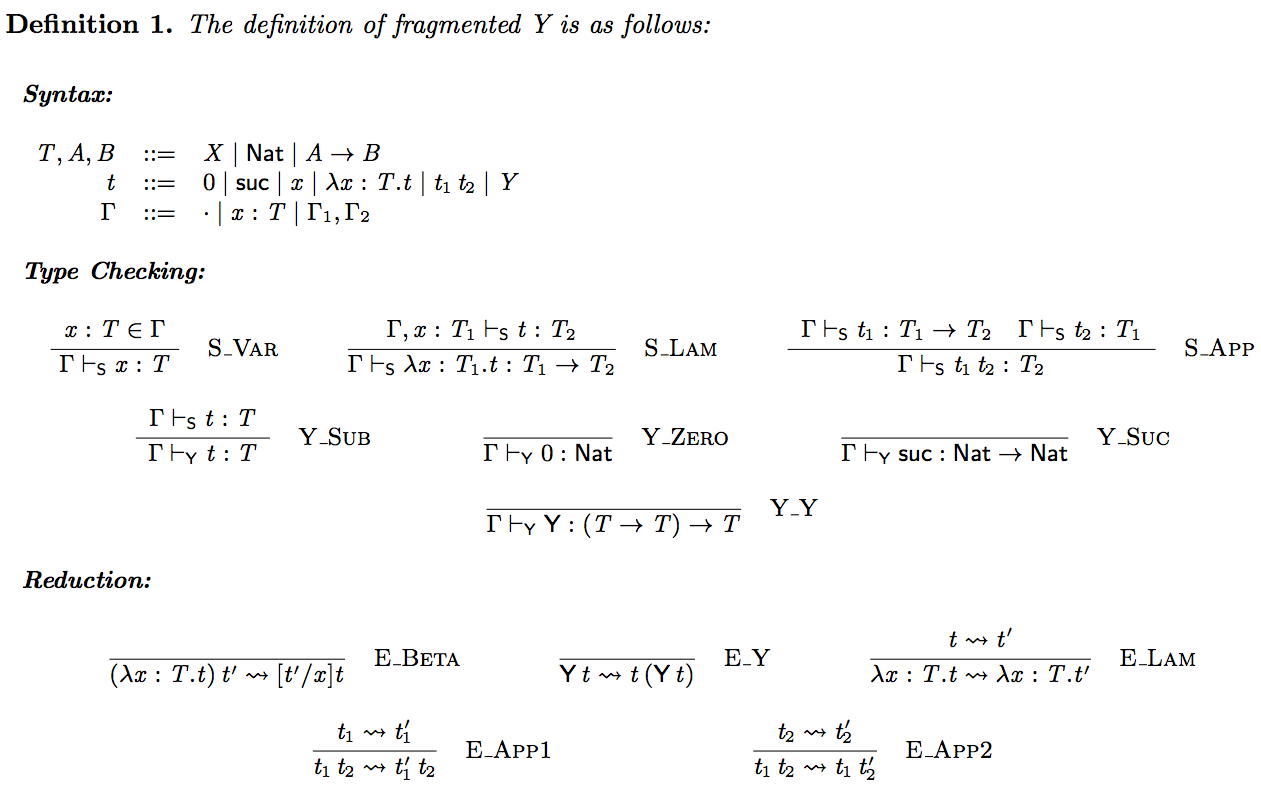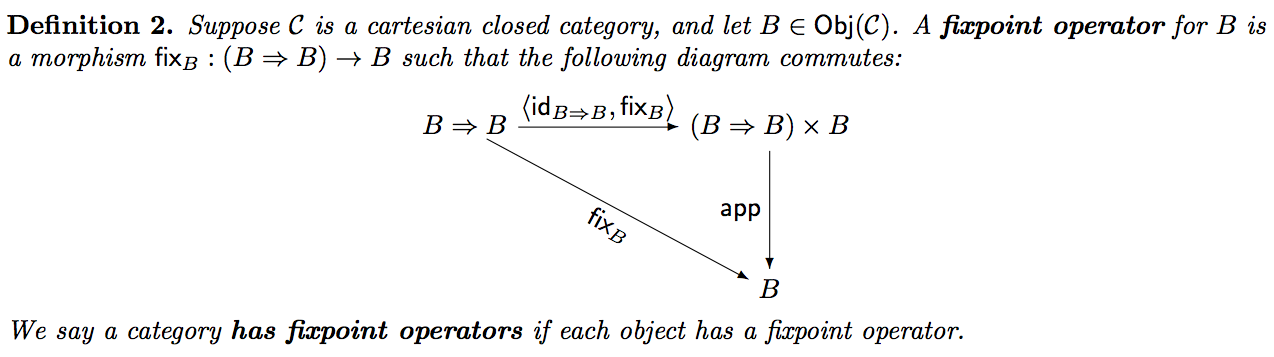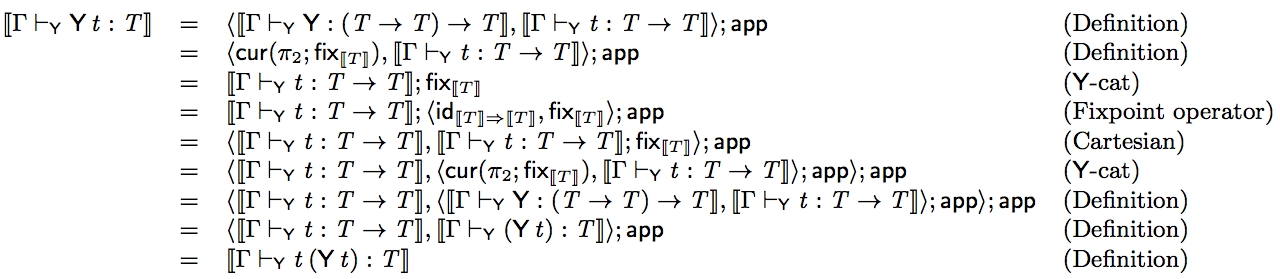## On the Categorical Model of Fragmented Y

Updated: 2014-12-01


I recently published a post on the categorical model of fragmented system T. Aaron Stump – my Ph.D. advisor – asked a very good question. One property the Trellys team wants in a PL is a non-terminating fragment to conduct general purpose programming. However, as Aaron pointed out fragmented system T has a terminating programmatic fragment, but what if we make it non-terminating? He asked particularly about adding the Y combinator. My response was that I did not know how to add just the Y combinator, but I argued that the programmatic fragment could be non-terminating by making it uni-typed.

I took his question as a challenge to figure out how to add just the Y combinator, and then obtain a categorical model. One thing had to be true of our model, it must have a cartesian closed subcategory to model the logical fragment in. Adding the Y combinator to STLC is simple enough, and since STLC is modeled by cartesian closed categories, then there should be an extension of cartesian closed categories with fixpoint operators. Keeping this idea in mind I did some thinking, and looked into the literature. It turns out that we can extend a cartesian closed category with fixpoint operators to obtain a model for the Y combinator. This post describes how this is done.

### Fragmented Y

First thing is first. We need to define the functional PL we will be analyzing.Just as we did in the previous post the programmatic fragment contains all typable terms using the rules annotated with , and the logical fragment contains all typable terms using the rules annotated with . We can easily see that the programmatic fragment is non-terminating. Clearly, $$_{} ,(x:T.x) : T$$, and
$$,(x:T.x) (x:T.x),(,(x:T.x)) ,(x:T.x)$$

In addition, it is well known that the logical fragment is terminating. Thus, the logical fragment is the isolation of a terminating subtheory of a non-terminating theory.

### Categorical Model

The idea is to argue how we can model the theory in a cartesian closed category with some additional structure. Just as we have said above the logical fragment can be modeled by a cartesian closed category. The subtheory rule $${}$$ can be modeled by functor from the model of the logical fragment to the model of the programmatic fragment. This is the same as it was for fragmented system T. In addition, it is easy to handle  and . So the most interesting question here is how do we model the programmatic fragment? The answer is well known, and can be found here – this book has a lot of great things in it, but lacks in presentation and the omission of details in some of the equational reasoning.

We model the programmatic fragment in a cartesian closed category extended with fixpoints for each object.In any cartesian closed category we have the morphism $$_2 : (T T) (T T)$$. Using this morphism we can define the following morphism in a cartesian closed category with fixpoint operators:
$$(_2;_T) : (T T) T$$
This morphism is the  combinator. That is, we can define
$$,=,(_2;_{})$$
A categorical model must have enough structure to model every typable term, but it must also model the reduction rules. That is, for any $$_{} t_1 : T$$ and $$_{} t_2 : T$$, if $$t_1 t_2$$, then $$=$$, where $${,}$$. It is well-known that if $$=$$, then the reduction rules of STLC can be modeled by a cartesian closed category. In fact, we have -equality in the model:
(-cat) $$(f),g ; = ,g ;((f) id); = ,g;f$$
Using -cat we can prove the following:We call the previous fact -cat. Now we can show that a cartesian closed category with fixpoint operators models the reduction rule for the -combinator:It took me the better part of the weekend to get the equational reasoning right, so hopefully I did not make any mistakes.

The way we model the Y combinator is slightly different from the book here. There the authors use the morphism $$!_{};(_2;_T) : (T T) T$$, where $$!_:$$ is the terminal morphism, but I do not see a reason to use the terminal morphism when we can just use $$_2$$. We obtain the same result here, but I might be missing something.

Finally, we arrive at the following main theorem:
Suppose that  is cartesian closed category (a model of the logical fragment of fragmented Y), and that  is a cartesian closed category with fixpoint operators (a model of the programmatic fragment of fragmented Y). Then $$(, S, )$$ is a model of fragmented Y, where $$S :$$ is a full and faithful functor.

It was a lot of fun figuring this out!